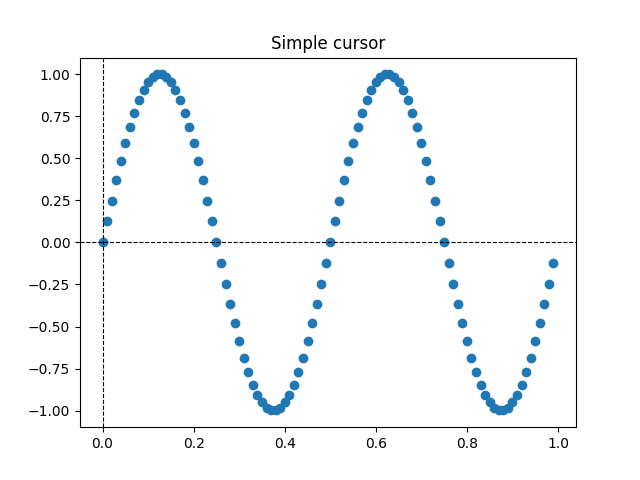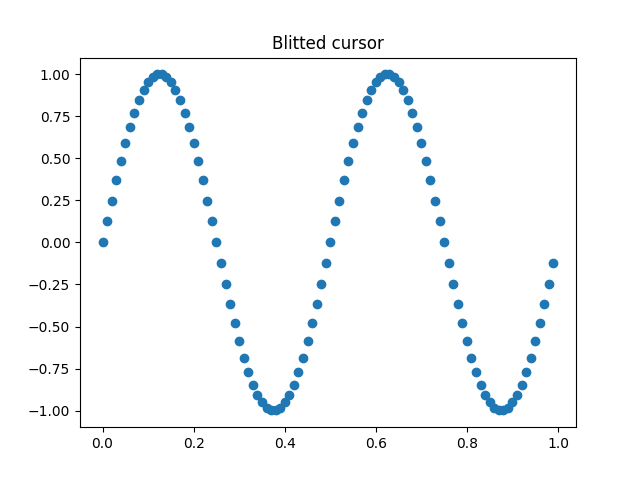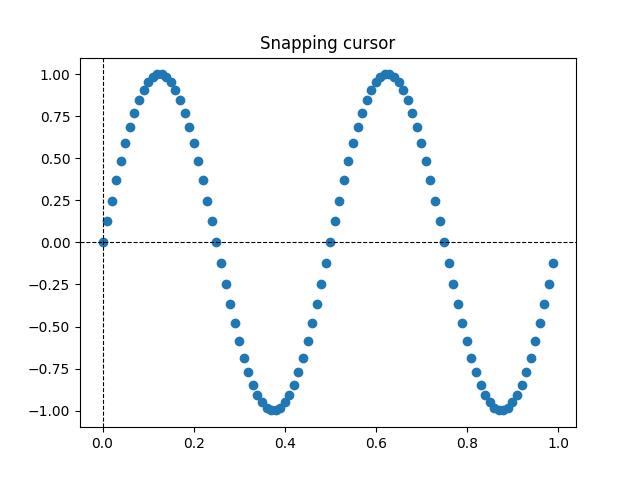# Cross hair cursor¶

This example adds a cross hair as a data cursor. The cross hair is implemented as regular line objects that are updated on mouse move.

We show three implementations:

1. A simple cursor implementation that redraws the figure on every mouse move. This is a bit slow and you may notice some lag of the cross hair movement.
2. A cursor that uses blitting for speedup of the rendering.
3. A cursor that snaps to data points.

Faster cursoring is possible using native GUI drawing, as in WXcursor Demo.

The mpldatacursor and mplcursors third-party packages can be used to achieve a similar effect.

import matplotlib.pyplot as plt
import numpy as np

class Cursor:
"""
A cross hair cursor.
"""
def __init__(self, ax):
self.ax = ax
self.horizontal_line = ax.axhline(color='k', lw=0.8, ls='--')
self.vertical_line = ax.axvline(color='k', lw=0.8, ls='--')
# text location in axes coordinates
self.text = ax.text(0.72, 0.9, '', transform=ax.transAxes)

def set_cross_hair_visible(self, visible):
need_redraw = self.horizontal_line.get_visible() != visible
self.horizontal_line.set_visible(visible)
self.vertical_line.set_visible(visible)
self.text.set_visible(visible)
return need_redraw

def on_mouse_move(self, event):
if not event.inaxes:
need_redraw = self.set_cross_hair_visible(False)
if need_redraw:
self.ax.figure.canvas.draw()
else:
self.set_cross_hair_visible(True)
x, y = event.xdata, event.ydata
# update the line positions
self.horizontal_line.set_ydata(y)
self.vertical_line.set_xdata(x)
self.text.set_text('x=%1.2f, y=%1.2f' % (x, y))
self.ax.figure.canvas.draw()

x = np.arange(0, 1, 0.01)
y = np.sin(2 * 2 * np.pi * x)

fig, ax = plt.subplots()
ax.set_title('Simple cursor')
ax.plot(x, y, 'o')
cursor = Cursor(ax)
fig.canvas.mpl_connect('motion_notify_event', cursor.on_mouse_move)Out:

5


## Faster redrawing using blitting¶

This technique stores the rendered plot as a background image. Only the changed artists (cross hair lines and text) are rendered anew. They are combined with the background using blitting.

This technique is significantly faster. It requires a bit more setup because the background has to be stored without the cross hair lines (see create_new_background()). Additionally, a new background has to be created whenever the figure changes. This is achieved by connecting to the 'draw_event'.

class BlittedCursor:
"""
A cross hair cursor using blitting for faster redraw.
"""
def __init__(self, ax):
self.ax = ax
self.background = None
self.horizontal_line = ax.axhline(color='k', lw=0.8, ls='--')
self.vertical_line = ax.axvline(color='k', lw=0.8, ls='--')
# text location in axes coordinates
self.text = ax.text(0.72, 0.9, '', transform=ax.transAxes)
self._creating_background = False
ax.figure.canvas.mpl_connect('draw_event', self.on_draw)

def on_draw(self, event):
self.create_new_background()

def set_cross_hair_visible(self, visible):
need_redraw = self.horizontal_line.get_visible() != visible
self.horizontal_line.set_visible(visible)
self.vertical_line.set_visible(visible)
self.text.set_visible(visible)
return need_redraw

def create_new_background(self):
if self._creating_background:
# discard calls triggered from within this function
return
self._creating_background = True
self.set_cross_hair_visible(False)
self.ax.figure.canvas.draw()
self.background = self.ax.figure.canvas.copy_from_bbox(self.ax.bbox)
self.set_cross_hair_visible(True)
self._creating_background = False

def on_mouse_move(self, event):
if self.background is None:
self.create_new_background()
if not event.inaxes:
need_redraw = self.set_cross_hair_visible(False)
if need_redraw:
self.ax.figure.canvas.restore_region(self.background)
self.ax.figure.canvas.blit(self.ax.bbox)
else:
self.set_cross_hair_visible(True)
# update the line positions
x, y = event.xdata, event.ydata
self.horizontal_line.set_ydata(y)
self.vertical_line.set_xdata(x)
self.text.set_text('x=%1.2f, y=%1.2f' % (x, y))

self.ax.figure.canvas.restore_region(self.background)
self.ax.draw_artist(self.horizontal_line)
self.ax.draw_artist(self.vertical_line)
self.ax.draw_artist(self.text)
self.ax.figure.canvas.blit(self.ax.bbox)

x = np.arange(0, 1, 0.01)
y = np.sin(2 * 2 * np.pi * x)

fig, ax = plt.subplots()
ax.set_title('Blitted cursor')
ax.plot(x, y, 'o')
blitted_cursor = BlittedCursor(ax)
fig.canvas.mpl_connect('motion_notify_event', blitted_cursor.on_mouse_move)Out:

6


## Snapping to data points¶

The following cursor snaps its position to the data points of a Line2D object.

To save unnecessary redraws, the index of the last indicated data point is saved in self._last_index. A redraw is only triggered when the mouse moves far enough so that another data point must be selected. This reduces the lag due to many redraws. Of course, blitting could still be added on top for additional speedup.

class SnappingCursor:
"""
A cross hair cursor that snaps to the data point of a line, which is
closest to the *x* position of the cursor.

For simplicity, this assumes that *x* values of the data are sorted.
"""
def __init__(self, ax, line):
self.ax = ax
self.horizontal_line = ax.axhline(color='k', lw=0.8, ls='--')
self.vertical_line = ax.axvline(color='k', lw=0.8, ls='--')
self.x, self.y = line.get_data()
self._last_index = None
# text location in axes coords
self.text = ax.text(0.72, 0.9, '', transform=ax.transAxes)

def set_cross_hair_visible(self, visible):
need_redraw = self.horizontal_line.get_visible() != visible
self.horizontal_line.set_visible(visible)
self.vertical_line.set_visible(visible)
self.text.set_visible(visible)
return need_redraw

def on_mouse_move(self, event):
if not event.inaxes:
self._last_index = None
need_redraw = self.set_cross_hair_visible(False)
if need_redraw:
self.ax.figure.canvas.draw()
else:
self.set_cross_hair_visible(True)
x, y = event.xdata, event.ydata
index = min(np.searchsorted(self.x, x), len(self.x) - 1)
if index == self._last_index:
return  # still on the same data point. Nothing to do.
self._last_index = index
x = self.x[index]
y = self.y[index]
# update the line positions
self.horizontal_line.set_ydata(y)
self.vertical_line.set_xdata(x)
self.text.set_text('x=%1.2f, y=%1.2f' % (x, y))
self.ax.figure.canvas.draw()

x = np.arange(0, 1, 0.01)
y = np.sin(2 * 2 * np.pi * x)

fig, ax = plt.subplots()
ax.set_title('Snapping cursor')
line, = ax.plot(x, y, 'o')
snap_cursor = SnappingCursor(ax, line)
fig.canvas.mpl_connect('motion_notify_event', snap_cursor.on_mouse_move)
plt.show()Keywords: matplotlib code example, codex, python plot, pyplot Gallery generated by Sphinx-Gallery